## Filters

Sort by :
Q
As we learnt in Mole Fraction - It is the ratio of moles of solute or moles of solvent to moles of the solution. - wherein If a substance ‘A’ dissolves in substance ‘B’ and their number of moles are  and  respectively; then the mole fractions of A and B are given as Mole fraction of A = (number of moles of A)/(number of moles of solution ) = /(+ )    At   ,                                      ...

a lone pair refers to a pair of valence electrons that are not shared with another atom and is sometimes called an unshared pair or non-bonding pair. Lone pairs are found in the outermost electron shell of atoms. They can be identified by using a Lewis structure.Engineering
375 Views   |

. An unstable heavy nucleus at rest breaks into two nuclei which move away with velocities in the ratio of 8 : 27. The ratio of the radii of the nuclei (assumed to be spherical) is :

• Option 1)

8 : 27

• Option 2)

4 : 9

• Option 3)

3 : 2

• Option 4)

2 : 3Option 1)

8 : 27

Option 2)

4 : 9

Option 3)

3 : 2

Option 4)

2 : 3

View More
Engineering
107 Views   |

Which of the following arrangements shows the schematic alignment of magnetic moments of antiferromagnetic substance?

• Option 1)• Option 2)• Option 3)• Option 4)As we learned

The substances which are expected to passes para-magnetism or ferromagnetism on the basis of unpaired electrons but actually, they possess zero net magnetic moments are called anti ferromagnetic substance.

Option 1)Option 2)Option 3)Option 4)View More
Engineering
72 Views   |

A man on the top of a vertical tower observes a car moving at a uniform speed
towards the tower on a horizontal road. If it takes 18 min. for the angle of
depression of the car to change from  to
; then after this, the time taken
(in min.) by the car to reach the foot of the
tower, is :

• Option 1)

• Option 2)

• Option 3)

• Option 4)

As we have learned

Angle of Elevation -

If an object is above the horizontal line from the eye, we have to raise our head to view the object.

- wherein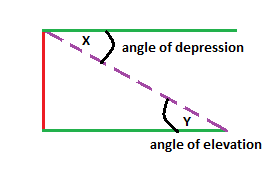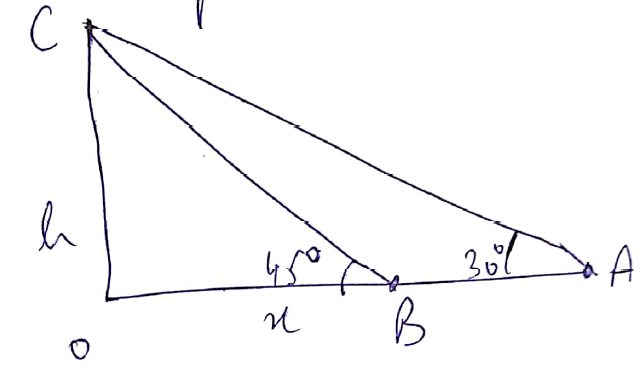Option 1)

This is correct

Option 2)

This is incorrect

Option 3)

This is incorrect

Option 4)

This is incorrect

View More
Engineering
453 Views   |

If a right circular cone, having maximum volume, is inscribed in a sphere of radius 3 cm, then the curved surface area (in cm2) of this cone is :

• Option 1)

• Option 2)

• Option 3)

• Option 4)

As we learned

Method for maxima or minima -

First and second derivative method :

- whereinr,h be the radius and height of sphere

in   ,using pythagorus theorem

So at    ; we get max  r=3   h = 4

thus

Option 1)

Option 2)

Option 3)

Option 4)

View More
Engineering
406 Views   |

An ideal capacitor of capacitance 0.2 μF is charged to a potential difference of 10 V. The charging battery is then
disconnected. The capacitor is then connected to an ideal inductor of self inductance 0.5 mH. The current at a time
when the potential difference across the capacitor is 5 V, is :

• Option 1)

0.34 A

• Option 2)

0.25 A

• Option 3)

0.17 A

• Option 4)

0.15 A

As we learned @3935   From energy conservation   Option 1) 0.34 A Option 2) 0.25 A Option 3) 0.17 A Option 4) 0.15 A
Engineering
826 Views   |

A force of 40 N acts on a point B at the
end of an L-shaped object, as shown in
the figure. The angle θ that will produce
maximum moment of the force about
point A is given by :• Option 1)

• Option 2)

• Option 3)

• Option 4)

As we learned

Torque -

- wherein

This can be calculated by using either

= perpendicular distance from origin to the line of force.

= component of force perpendicular to line joining force.

for maximum torque

Option 1)

Option 2)

Option 3)

Option 4)

View More
Engineering
144 Views   |

If ,  then the value of  at the point (-2,0) is :

• Option 1)

• Option 2)

• Option 3)

• Option 4)

As we learned

Derivative at a point -

The value of  f'(x) obtained by putting  x = a is called the derivative of  f(x) at  x = a  and it is denoted by  f'(a)  or

at  x = a.

-

On Differentiating , we get

at (-2,0)

at (-2,0)

Option 1)

Option 2)

Option 3)

Option 4)

View More
Engineering
731 Views   |

It is found that if a neutron suffers an elastic collinear collision with deuterium at rest, fractional loss of its energy is pd ; while for its similar collision with carbon nucleus at rest, fractional loss of energy is pc. The values of pd and pc are respectively :

• Option 1)

(0, 1)

• Option 2)

(⋅89, ⋅28)

• Option 3)

(⋅28, ⋅89)

• Option 4)

(0, 0)

Perfectly Elastic Collision -

Law of conservation of momentum and that of Kinetic Energy hold good.

- wherein

Before Collision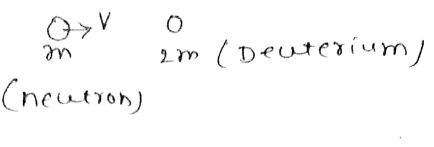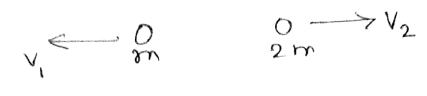for elastic collision :

For collision with carbon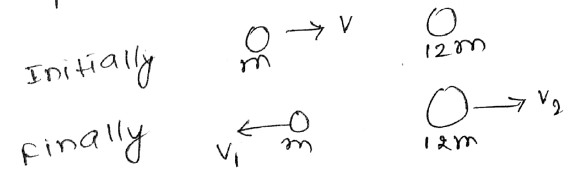Fraction Loss =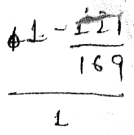Option 1)

(0, 1)

Option 2)

(⋅89, ⋅28)

Option 3)

(⋅28, ⋅89)

Option 4)

(0, 0)

View More
Engineering
97 Views   |

If , then  equals :

• Option 1)

• Option 2)

• Option 3)

• Option 4)

As we learned,

Option 1)

Option 2)

Option 3)

Option 4)

View More
Engineering
102 Views   |

Let  and  Then,
the least odd natural number p, so that , for all , is:

• Option 1)

9

• Option 2)

7

• Option 3)

11

• Option 4)

5

As we learned,     If  i.e.   Thus least value of p=7 Option 1) 9 Option 2) 7 Option 3) 11 Option 4) 5
Engineering
101 Views   |

Let  be a function defined as  where  and . Then f is :

• Option 1)

invertible and

• Option 2)

invertible and

• Option 3)

invertible and

• Option 4)

not invertible

As we learned,

The function is invertible and

Option 1)

invertible and

Option 2)

invertible and

Option 3)

invertible and

Option 4)

not invertible

View More
Engineering
870 Views   |

The characteristic distance at which quantum gravitational effects are significant, the Planck length, can be determined from a suitable combination of the fundamental physical constants G, h and c. Which of the following correctly gives the Planck length ?

• Option 1)

• Option 2)

• Option 3)

• Option 4)

As we have learnt @1035

let planck length is

Planck Length =

Option 1)

Option 2)

Option 3)

Option 4)

View More
Engineering
776 Views   |

In a screw gauge, 5 complete rotations of the screw cause it to move a linear distance of 0.25 cm. There are 100 circular scale
divisions. The thickness of a wire measured by this screw gauge gives a reading of 4 main scale divisions and 30 circular scale divisions. Assuming negligible zero error, the thickness of the wire is :

• Option 1)

0.4300 cm

• Option 2)

0.2150 cm

• Option 3)

0.3150 cm

• Option 4)

0.0430 cm

As we learned

To measure the diameter of small spherical cylindrical body using Vernier Callipers -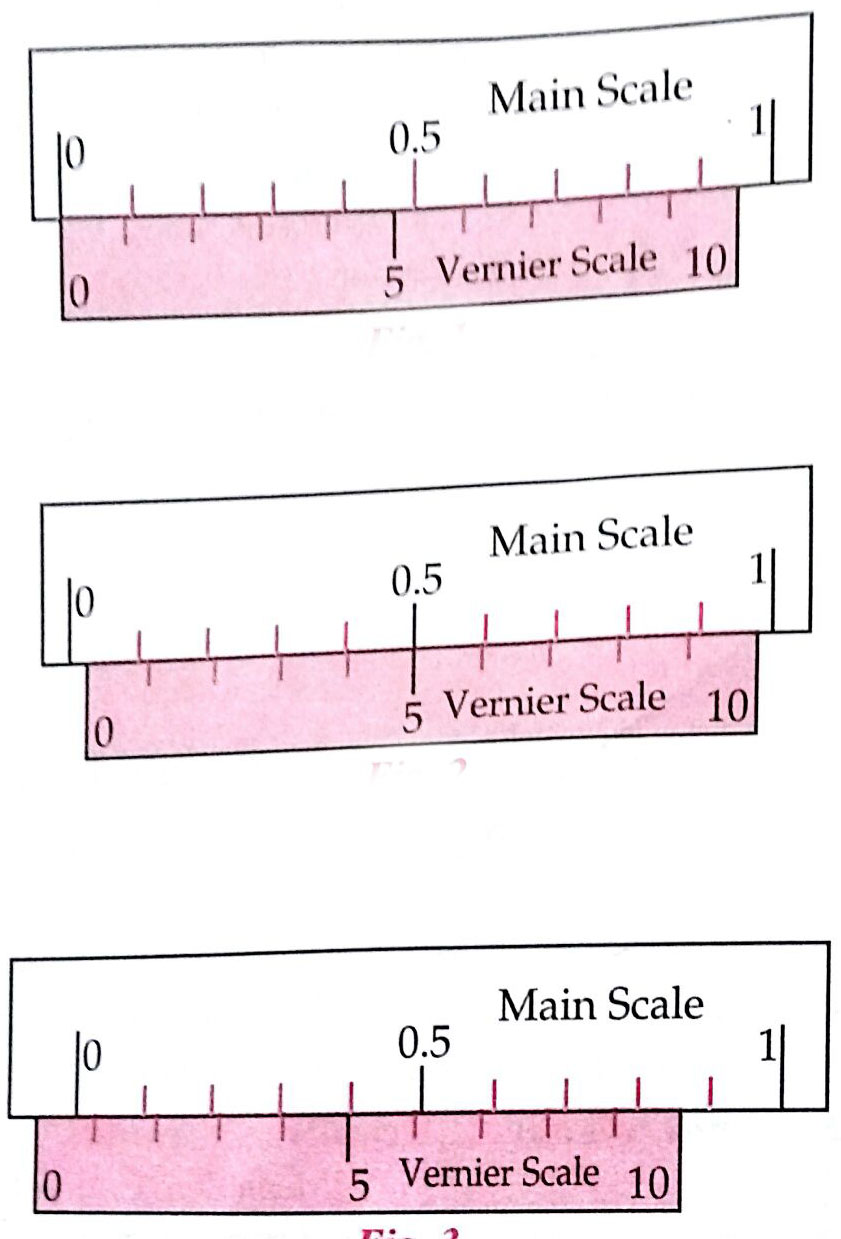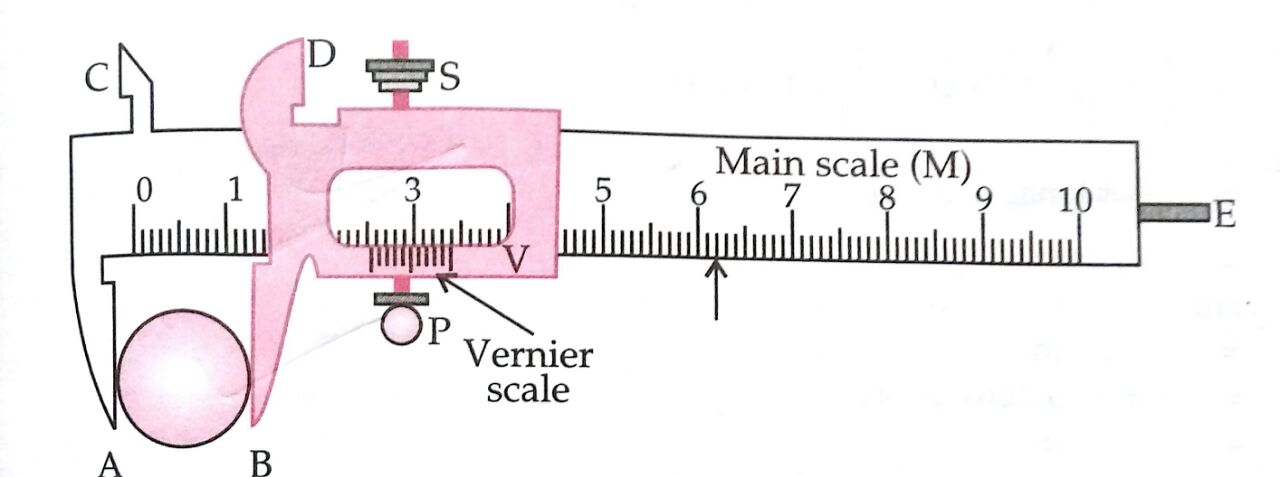Vernier Constant

= 1 Main scale division - 1 V.S. Division

V.C= 1 M.S.D - 1 V.S.D

- wherein

N= Nth division

Observations:

1.    Vernier constant (least count) of the Vernier Callipers:

1 M.S.D. = 1 mm

10 vernier scale divisions = 9 main scale divisions

i.e.     10 V.S.D. = 9 M.S.D.

1 V.S.D. =  M.S.D.

Vernier Constant (L.C.) = 1 M.S.D. - 1 V.S.D. = 1 M.S.D.  M.S.D.

2.    Zero error: (i) ........ cm (ii) ............cm (iii) ..........cm

Mean Zero Error (e) = ............ cm

Mean Zero Correction (c) = - (Mean Zero Error)

= .......... cm

= 0.2150cm

Option 1)

0.4300 cm

Option 2)

0.2150 cm

Option 3)

0.3150 cm

Option 4)

0.0430 cm

View More
Engineering
425 Views   |

A uniform rod AB is suspended from a point X, at a variable distance x from A, as shown. To make the rod horizontal, a mass m is suspended from its end A. A set of (m, x) values is recorded. The appropriate variables that give a straight line, when plotted, are :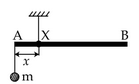• Option 1)

m, x

• Option 2)

• Option 3)

• Option 4)

As we learned

Torque -

- wherein

This can be calculated by using either

= perpendicular distance from origin to the line of force.

= component of force perpendicular to line joining force.

Balancing Torque w.r.t point of suspension

This represents a straight line

Option 1)

m, x

Option 2)

Option 3)

Option 4)

View More
Engineering
146 Views   |

The number of amplitude modulated broadcast stations that can be accomodated in a 300 kHz band width for the highest modulating frequency 15 kHz will be :

• Option 1)

20

• Option 2)

15

• Option 3)

10

• Option 4)

8

As we learned

Band width -

It is frequency range over which an equipment operate or the portion of the spectrum occupied by the signal.

-

Frequency required for one station

Number of channel = 10

Option 1)

20

Option 2)

15

Option 3)

10

Option 4)

8

View More
Engineering
110 Views   |

In a common emitter configuration with suitable bias, it is given that  is the load resistance and  is small signal dynamic
resistance (input side). Then, voltage gain, current gain and power gain are given, respectively, by : β is current gain, ,  and  arerespectively base, collector and emitter currents.

• Option 1)

• Option 2)

• Option 3)

• Option 4)

As we learned

Relation between α and β -

- wherein

(current gain )

Current Gain

Vol + age Gain =

power gain = (voltage gain) (Current gain)

Option 1)

Option 2)

Option 3)

Option 4)

View More
Exams
Articles
Questions